## PIP

 Creates a picture-in-picture effect.

 USAGE: pip [-s size] [-p points] [-a angle] [-t thickness] [-f framecolor] [-d darkness] [-r rolloff] [-e extent] [-B brightness] [-S saturation] [-h hue] [-C contrast] [infile] [outfile] USAGE: pip [-help] -s ... size ......... size of frame; if one value is specified, it will be used as ..................... a percentage of the dimensions of the input image; if two ..................... values are specified as WxH, then those dimensions will be used ..................... for the Width and Height of the frame; values are integers>0; ..................... default=60 -p ... points ....... points is up to two pair of X,Y coordinates within the image; ..................... if no points are specified (default), then the center of the ..................... input image will be computed and use for the center of the frame; ..................... if one point is specified, it will be used for the center of ..................... frame; if two points are specified, they will be interpreted ..................... as defining a diagonal of the frame. Values are integers>=0 -a ... angle ........ angle of rotation of the frame in degrees; angle are positive ..................... in the clockwise direction and negative in the counterclockwise ..................... direction; values are integers; default=-15 -t ... thickness .... thickness of the frame; values are integers>0; default=10 -f ... framecolor ... framecolor is the color of the frame; any valid IM color is ..................... allowed; default=white -d ... darkeness .... darkeness of the frame shadow; 0<=integer<=100; default=60 -r ... rolloff ...... rolloff of the shadow darkness; integer>=0; default=3 -e ... extent ....... extent (distance) of the shadow; integer>=0; default=5 -B ... brightness ... brightness percent change; -100<=integer<=100; default=0 -S ... saturation ... saturation percent change; -100<=integer<=100; default=0 -H ... hue .......... hue angle change in degrees; -360<=integer<=360; default=0 -C ... contrast ..... sigmoidal contrast change; values are integers; default=0 . PURPOSE: To create a picture-in-picture effect. DESCRIPTION: PIP creates a picture-in-picture effect. The script adds a picture frame inside the image to highlight some region of interest in the image. ARGUMENTS: -s size ... SIZE of frame. If one value is specified, then it will be used as a percentage of the dimensions of the input image. If two values are specified as WxH, then those dimensions will be used for the Width and Height of the frame. Values are integers>0. The default=60 -p points ... POINTS is up to two pair of X,Y coordinates within the image. If no points are specified (default), then the center of the input image will be computed and use for the center of the frame. If one point is specified, it will be used for the center of frame. If two points are specified, they will be interpreted as defining a diagonal of the frame. Values are integers>=0. The default is to compute the center of the image as the frame center. -a angle ... ANGLE of rotation of the frame in degrees. Angles are positive in the clockwise direction and negative in the counterclockwise direction. Values are -90<=integers<=90. The default=-15. -t thickness ... THICKNESS of the frame. Values are integers>0. The default=10. -f framecolor ... FRAMECOLOR is the color of the frame. Any valid IM color is allowed, even partially transparent colors. The default=white. -d darkeness ... DARKNESS of the frame shadow. Values are 0<=integer<=100. The default=60. -r rolloff ... ROLLOFF (fading) of the shadow darkness. Values are integer>=0. The default=3. -e extent ... EXTENT (distance) of the shadow. Values are integer>=0; default=5. -B brightness ... BRIGHTNESS percent change of the image. Value are -100<=integer<=100. The default=0. -S saturation ... SATURATION percent change of the image. Value are -100<=integer<=100. The default=0. -H hue ... HUE angle change of the image in degrees. Value are -360<=integer<=360. The default=0. -C contrast ... CONTRAST change in the image. Value are positive or negative integers. The default=0. This is a sigmoidal-contrast adjustment so values are typically 0 to 10. CAVEAT: No guarantee that this script will work on all platforms, nor that trapping of inconsistent parameters is complete and foolproof. Use At Your Own Risk.

### EXAMPLES

 Example 1 Original Image (source)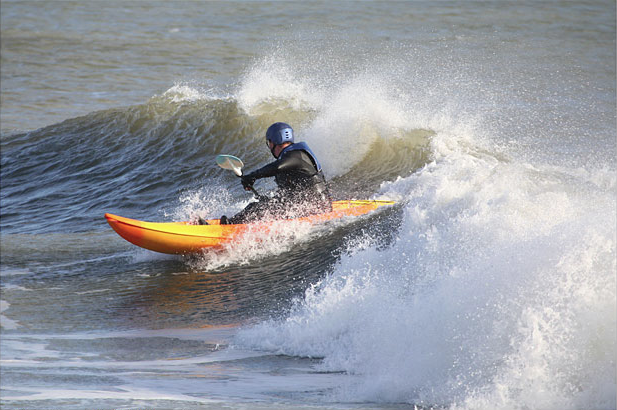Arguments: (scale and center) -s 60 -p "265,185" -a -15Arguments: (dimensions and center) -s "400x250" -p "265,185" -a -15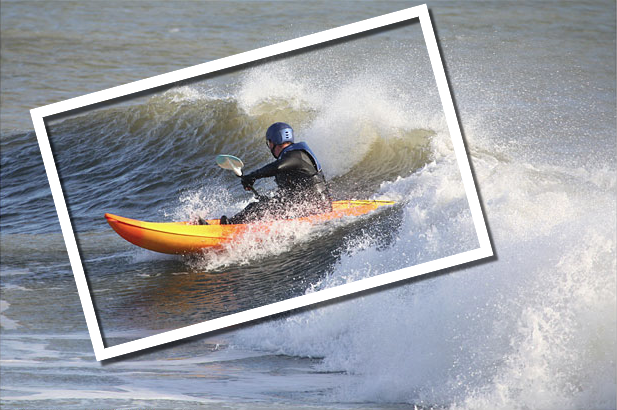Arguments: (scale and diagonal end points) -s 60 -p "59,115 464,248" -a -15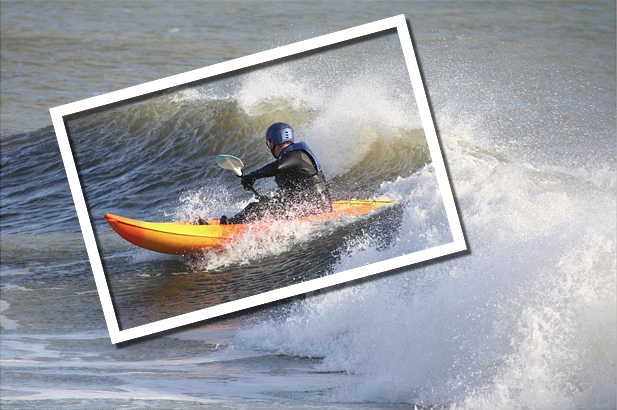Example 2 Original Image (source)Arguments: -s 60 -p "320,220" -a -15Example 3 Original Image (source)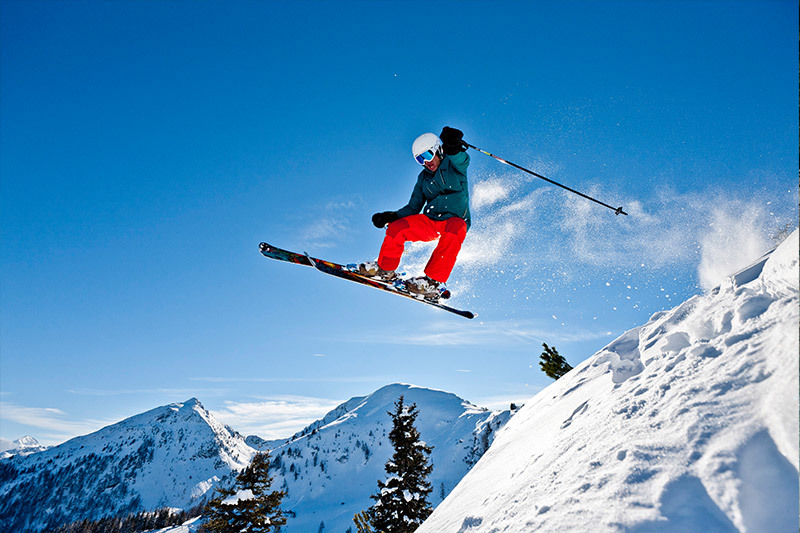Arguments: -s 55 -p "425,230" -a -20 -f white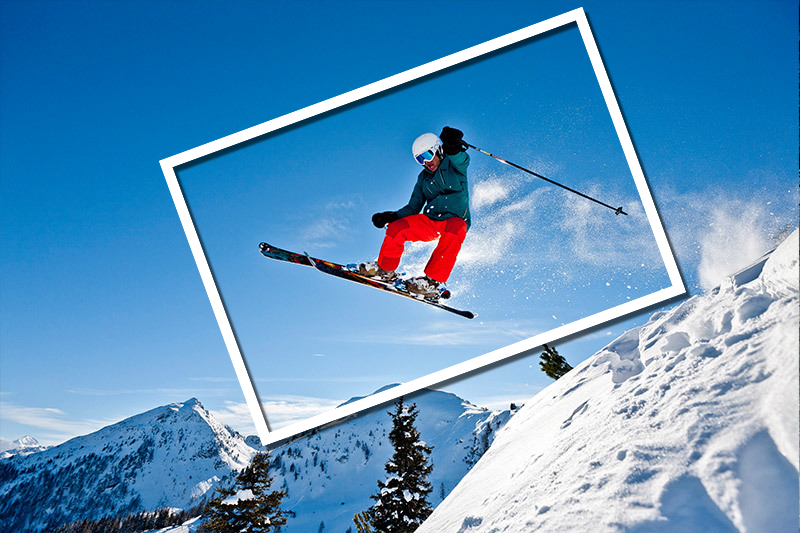Arguments: -s 55 -p "425,230" -a -20 -f pink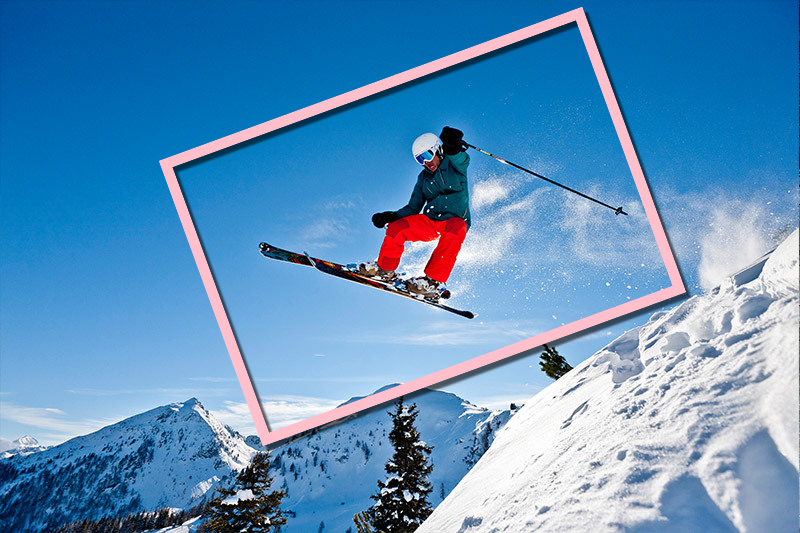Example 4 Original Image (source)Arguments: (default to center of image) -s 70 -a -10 -f whiteArguments: (default to center of image) -s 70 -a -10 -f "#ff000077"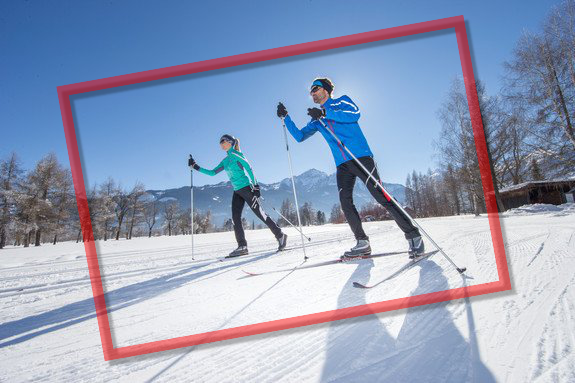What the script does is as follows for the scale and centering case: Computes the frame dimensions and location from the scale and point (center) arguments Draws a frame on a transparent image the size of the input image Adds a shadow to the frame )ptionally applies brightness, saturation, hue and/or contrast adjustment to the image Merges the shadowed frame image onto the modified input image ww=`convert -ping "\$infile" -format "%w" info:` hh=`convert -ping "\$infile" -format "%h" info:` cx=`echo "\${points}," | cut -d, -f1` cy=`echo "\${points}," | cut -d, -f2` center="\$cx,\$cy" wd=`convert xc: -format "%[fx:\$size*\$ww/100]" info:` ht=`convert xc: -format "%[fx:\$size*\$hh/100]" info:` wd2=`convert xc: -format "%[fx:\$wd/2]" info:` ht2=`convert xc: -format "%[fx:\$ht/2]" info:` bri=\$((100+\$brightness)) sat=\$((100+\$saturation)) hue=`convert xc: -format "%[fx:(200/360)*\$hue+100]" info:` sign=`convert xc: -format "%[fx:sign(\$contrast)>=0?1:0]" info:` con=`convert xc: -format "%[fx:abs(\$contrast)]" info:` convert -size \${ww}x\${hh} xc:none -stroke \$framecolor -strokewidth \$thickness -gravity center \ -fill none -draw "translate \$center rotate \$angle rectangle -\$wd2,-\$ht2 \$wd2,\$ht2" \ \( +clone -background black -shadow \${darkness}x\${range}+\${extent}+\${extent} \) \ +swap -background none -layers merge +repage \ \( "\$infile" \$sigmoidal -modulate \$bri,\$sat,\$hue \) +swap -compose over -composite \ "\$outfile"